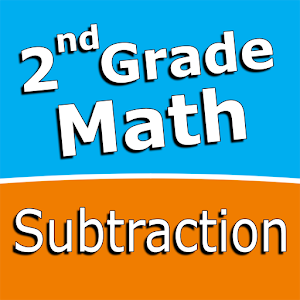Türkçe

Her gün ücretsiz lisanslı Android uygulamaları ve oyunlar sunuyoruz.\$2.99
SÜRESI DOLMUŞ

# Android Giveaway of the Day - Second grade Math - Subtraction

Intuitive interface powered by handwriting input and a fun and engaging mini game.
\$2.99 SÜRESI DOLMUŞ
Kullanıcı puanı: 1 (100%) 0 (0%)

Bu programın süresi doldu. Second grade Math - Subtraction artık firmadan temin edilebilir.

Intuitive interface powered by handwriting input and a fun and engaging mini game in addition to a regular math trainer mode make our app stand out from the crowd of generic math learning apps.

With Second grade Math - Subtraction you can practice and improve the following math skills:
- Subtraction facts - numbers up to 20
- Subtract a one-digit number from a two-digit number
- Subtract two two-digit numbers
- Subtract multiples of 10
- Subtract multiples of 100
- Subtract multiples of 10 or 100
- Subtract three-digit numbers
- Balance subtraction equations

Sergey Malugin

Education

### Sürüm:

Varies with device

### Boyut:

Varies with device

Everyone

### Uyumluluk:

Varies with device

## Second grade Math - Subtraction üzerine yorumlar

Thank you for voting!